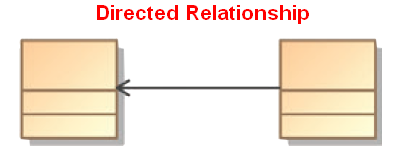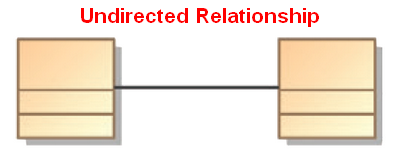# Logical Data Modeling - Reflexive relationship property

reflexive is a relationship property that indicates:

• that the relationship relates every element to itself.
• in other word, that the relation set representing the relationship contains every tuple:
• in a binary relationship - the relation set contains all <a,b> such as a=b
• in a N relationship: <a,b,c..., n> such as a=b=c…=n

## Mathematical Definition

A binary relation Rel is called reflexive:

• on a a dimensional set A, if the relation set contains every tuple <a, b> for every element a of A.

$$\{(a)\in\Bbb A\mid a = a\} \in Rel$$

## Example

### Less than or equal

The relation less than or equal to (<=) on the set of integers {1, 2, 3} is the following set of tuple

<1, 1>,
<1, 2>,
<1, 3>,
<2, 2>,
<2, 3>,
<3, 3>



It is reflexive because the tuples <1, 1>, <2, 2>, <3, 3> are in this relation.

As a matter of fact, this relation is reflexive on any set of numbers (not only integer but also real numbers, …).

Against the $R$ , the relation less than or equal to is the below gray area in $R^2$

### Equivalence relationship

Similarly and because every number is equal to itself, the relation greater than or equal and is equal to on any set of numbers are reflexive.

### Plot

A few relations:

• between x and y
• on subsets of $R$ (real number)
• represented as subsets of $R^2$

The gray area is the relation.

In the set of real number, a relation is reflexive if it contains the dotted line y=x

Example:

• Because the line y=x is in the relation, this is then a reflexive relation (but it's not symmetric, nor antisymmetric)
• Because the line y=x is in the relation, this is a reflexive relation (and it's symmetric)
• Because the line y=x is partially in relation, this is NOT a reflexive relation (nor symmetric or antisymmetric )
• Because the line y=x is not in the relation, this is then NOT a reflexive relation (but it's an antisymmetric)

## Documentation / Reference

Recommended PagesLogical Data Modeling - Antisymmetry relationship

A Antisymmetric relation is a relationship that happens when for all a and b in X: if a is related to b then b isNOT related to a or b=a (reflexivity is allowed) In mathematical notation, an Antisymmetric...Logical Data Modeling - Asymmetric Relation (Uni-directional|Antisymmetric)

An asymmetric relation is a type of binary relation that requiers: antisymmetry (ie if a is related to b, b is not related to a) and irreflexivity (ie an element cannot be related to itself) irreflexivity...Logical Data Modeling - Binary Relation

A binary relation is a relationship between two elements that is implemented via a binary function. Binary relations are used in many branches of mathematics to model concepts like: order relation...Logical Data Modeling - Equivalence Relationship (Equality)

The equivalence relation is a relationship between two entities. The equivalence relation is also known as: is equal to. equality is parallel to (for affine spaces) is in bijection with - bijection...Logical Data Modeling - Relationship

A relationship is a model element that relates two entities . In its simple form (semantic form), it's a description that has a verb such as: has consists of uses ... entityentity setrelationship...Logical Data Modeling - Symmetric relationship (bi-directional)

A symmetric relation is a type of binary relation that happens when for all a and b in X if a is related to b, then b is related to a In mathematical notation, the relation f between x and y is...Logical Data Modeling - Transitive Relationship Property

transitive is a relationship property that tells that the relationship follows the following rule: when the relation relates a to b and b to c, then the relation relates a to c. In mathematical notation,...Ordinal Data - Partially Order

A Partially ordered structure is a data structure where not every pair of elements needs to be comparable (compared). A collection of people ordered by genealogical descendancy: Some pairs of...